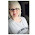## Wednesday, January 1, 2014

### Team 49 - Kimes **

I am from a small town in Southeast Nebraska. My name is Janette Kimes . My horse this year will be Bella, a 7 year old Quarter Horse,   And Truck a 14 year old Quarter Horse. Bella will be my  mount most of the time. Since I was a  little kid I have  been riding for the joy of it. To me riding has to be the most peaceful, relaxing thing in the world.
I have been in the derby for 2 years, The first year I came in 4th with 1346 miles . It was fun but more of a job than relaxing. The 2nd year { do to a wreck my horse and I had } I didn't ride  much after July.  I came in with just a few miles over 500. This year I will try to be somewhere in the middle.
We take our horses  and go camping with them a lot, One of my favorites Indian Cave for a hard ride, and Rock  Creek St. for a more relaxing ride. I live less than a hour drive from both, right in the middle. Lucky.
~~~~~~~~~~~~~~~~~~~~~~~~~~~~~~~~~~~~~
2012 / 1346.93 Miles / 4th Place
2013 /  503.34 Miles /  35th Place

1.Jan-18 2:45
Jan-19 4:50
that=7:35

2.I think this should have be 6.95 :-)

3.Mar, 06 =4:50
Mar,09 = 5:10
Mar.10= 8:50
that =25:45

4.Mar. 12 =2:15
mar.14- 5:20
Mar. 15- 17:50
YTD =42:95

5.Mar. 16-3:12
YTD=46:07

6.My spreadsheet has you at 53.02 YTD after adding all your miles.

7.Ok thanks I guess I can not add.

8.Mar.18 - 7:23
YTD =60:25

9.Mar. 20- 5:90
YTD =66:15

10.Mar.21-3:40
YTD -69:55

11.Mar. 26- 4:22
Mar. 28-12:73
YTD =88:50

12.Mar.29- 18:10
YTD=106:60

13.Congrats on getting over 100 miles :-)

14.June 3=5:40
june 5= 4:10
June 6=11:76
YTD 127:86

15.June 6= 10:10 this the 2nd ride I had today, rode by my self this morning, them this afternoon with friends.
YTD=137:96

16.It was a great day for riding, I rode both my horses tonight after work. FUN FUN
June 10=5:16 on Bella
June 10=4:22 on Truck
YTD =147:44

17.June 11=3:34 on Bella
YTD=150:78

18.June 12=5:22
June 14=4:37
June 16=9:78
June 17=10:12
YTD =180:27

19.June=22 = 7:90
YTD =188:17

20.I have been writing the wrong month sorry
April-30 =5:23
May- 01 =2:10
May-02 =6:78
YTD =202:28

21.May3=8:12
May5=4:46
May6= 9:12
YTD =223:98

22.May 9=4:76
May10=17:12
YTD=245:86

23.May12=2:90
May13=6:21
May14=9:35
YTD +264:32

24.May16=4:34
May17=12:43
YTD=281:09

25.May 18= 7:30
May 20=12:56
YTD =300:95

26.Congrats on getting over the 300 mile mark!

27.May 22=5:12
YTD=306:07

28.May24=2:23
May26=8:90
May27=11:10
YTD=328:30

29.May28=2:10
May30=9:22
YTD =339:62

30.June 1=4:20
June 2=5:32
YTD=349:14

31.June 3=4:90
June 4=8:02
YTD==362:06

32.June 9= 1:30
June10=5:10 Morning
June 10=12:50
YTD=380:96

33.June 11=15:10
YTD=396:06

34.June 12=2:20
June 14=18:30
YTD=416:56
We went to Indian Cave for the day Sat. It was great. This year they have inproved the trails 100% great ride.

35.Congrats on getting over 400 miles :-)

36.June 15=1:60
June 16=5:30
June 18=4:20
June 19=3:10
YTD=430:76

37.June 20=2:50
June 21=13:90
YTD=447:76

38.June 22= 3:10
June 23=4:20
YTD =455:06

39.June 24=8:60
YTD=463:66

40.June 26=7:10
june 28=6:50
YTD=477:26

41.June29=9:50
June30=1:20
July1=14:30
YTD=502:26

42.congrats on getting over 500 miles :-)

43.July3=6:20
July5=18:40 Went to Rock Creek, Fun Fun Fun
YTD=526:86

44.July 7=7:90
YTD=534:90

45.July11=4:90
July13=7:10
YTD=546:90

46.July15=11:20
July16=1:10
YTD=559:20

47.Hey Janette - Just checking up on you. Everything okay? Haven't seen any miles lately - Tammy

48.Sept. 9 =5:40
Sept.11=7:10
sept.12=15:60
YTD=587.30
I am riding a different hors now ,My spare horse is now my riden horse. Truck is a good boy and is enjoying to time on the rode.

49.Sept.14= 5:60
YTD=592;90

50.Sept. 16=2:40
Sept 18 =8:20
YTD =603:50

51.Sept 19=2:10
Sept 20=12:50
YTD= 618:10

52.Congrats on getting over 600 miles :-)

53.Sept 22= 5:50
Sept25= 2:80
Sept26=5:10
Sept 27=7:30
Sept 28=15:60
Ytd=654:40

54.Oct. 2 =4:20
Oct 3=6;70
Oct 5=11:90
YTD =677:20

55.Oct. 6= 5:30
Oct 7= 4:90
Oct 8= 9:80
YTD =697:20

56.Oct. 10=9:10
oct 11= 8:50
YTD = 714:80

57.Congrats on getting over 700 miles :-)

58.Oct 13=7:10
Oct 15=5:40
Oct16= 2:70
Oct17=7:20
YTD =737:20

59.Oct18= 15:90
YTD=753:10

60.Oct 20=4:90
Oct 23=6:30
Oct 24= 5:20
Oct 25=14:90
oct 26=18;10
YTD=802:50

61.Congrats on getting over 800 miles :-)

62.oct24=n8:60
oct25=2:70
Oct27=3:10
oct30=7:20
YTD=824:10

63.oct 31=9:70
YTD=833:80